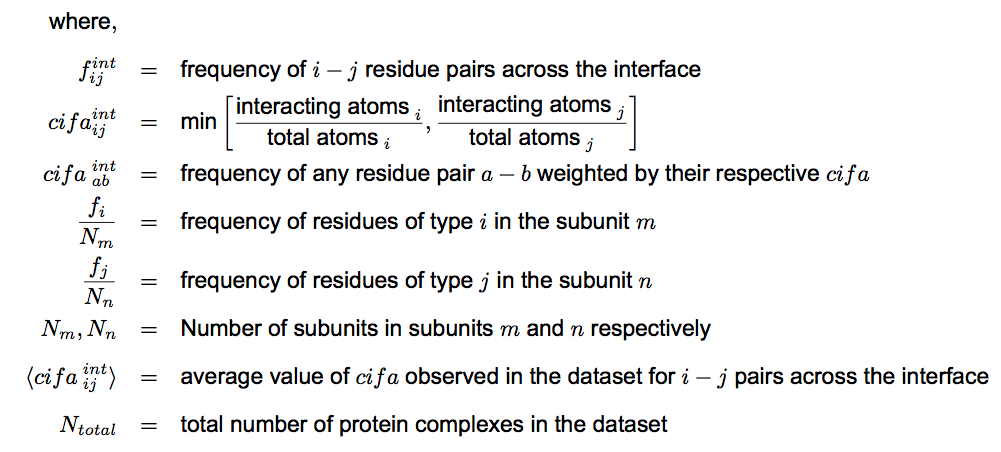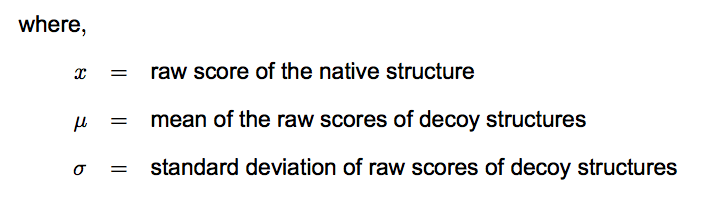Interacting amino acid residues
Two amino acid residues are defined as interacting if any relevant atom of residue A of type i is within the distance threshold of any relevant atom of residue B of type j.
Scores file
This is the main results file that contains the prediction along with the parameters used.
Raw Score
The raw score of a given interface is the sum of the individual scores of amino acid pairs that make up the interface. Each amino acid residue pair was assigned a score based on the statistical likelihood of that pair being a part of a native interface. The exact formulation of the scoring function isZ-score
For each interface, a Z-score is computed to assess the significance of the raw score. The Z-score is an estimate of the likelihood that the native interface is different than a decoy interface (in our case, random associations between the interface residues).Where,
The ‘Average Background Score’ and ‘Standard Deviation’ reported in the scores file represent and used to compute the Z-score.
Each statistical potential used in this server has its corresponding Z-score threshold that was estimated using Receiver-Operator curves to classify an interface as binding. More negative Z-scores imply a more significant likelihood.
Mutational Analysis Score
The native structure is scored according to the potential each residue on the interface is mutated to alanine (or to every other residue, depending on the type of option selected by the user) and the score of the resulting mutant complex is calculated.

The difference between the scores of the native structure and the score of the mutant structure becomes the 'Score-Diff' for the residue which was mutated.
Average Background Score and Standard Deviation
For each query structure that is submitted to PIZSA, we create 1000 decoy structures to test the statistical significance of the raw score computed for the query structure. Each of these 1000 decoys are in-turn scored by the same potential and their scores constitute 'the background scores set'. The average background score is the mean of the scores in the background set (the score of a decoy on average) whereas, the standard deviation describes the spread of the decoy scores around the average decoy score.

Z-score ((Native raw score - mean decoy score) / standard_deviation_decoyset))